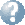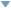Online Reference Dictionary, Encyclopedia & moreby: WordStarts with Ends withMentionsteaching:topics:calculus:sequences

### Sums of Sequences

These are called ‘Series’ … a very important concept in dealing with motion, physics and much more.

Can we possibly get a sensible, finite, answer when we try to add up an infinite number of positive numbers all greater than zero?

This is a very ancient problem in mathematics — the different ways of resolving (or banishing) it have been profoundly important to mathematics, physics and wider ideas of philosophy. And to the history of the development of modern science, technology and industry along with modern economies and societies.

Consider a simple question like this:

A wolf is chasing an Olympic champion.
The wolf is running twice as fast as the man.
Can the wolf ever catch him?

First the wolf runs along and reaches the place where the man has just been. But the man has run on himself … he is still safely ahead of the wolf. So the wolf keeps running until he reaches the man’s new position. Again, when it reaches that position the man has run further and is still in front.

Clearly the wolf can repeat this effort over and over again but every time the man will have run a bit further. This attempt to reach the man will continue endlessly.

So the wolf will never catch up.

but equally clearly this is nonsense — there must be something wrong with the argument — we know well that the wolf will catch up soon enough.

Say the man has a 100m head start, and since he is an olympic sprinter he can run 10m/s. When and where will the wolf catch him?

Now think about the answer described above … how many metres does the wolf run in each interval? $100, 50,25,12.5 \dots\qquad\text{or}\qquad w_n=\frac{200}{2^n}$ … here we are using w_n to mean the number of meters the wolf runs during the $n$th period described in that answer. How many seconds in each of these intervals? $5,2.5,1.25\dots\qquad\text{or}\qquad t_n=10\times\frac{1}{2^n}$

These sets of numbers are called ‘Sequences’ … now lets think about writing an equation for the total distance the wolf has travelled after 5 of these intervals (we will call that distance W_5) … $W_5 = 100 + 50 + 25 + \textstyle 12\frac{1}{2} + 6\frac{1}{4}$ after $n$ intervals … \begin{align} W_n &= w_1 + w_2 + w_3 +\cdots+ w_n\\ \\ &= 100 + 50 + \frac{200}{8}+\frac{200}{2^4}+\frac{200}{2^5}+\cdots+ \frac{200}{2^n} \end{align}

These sums of Sequences we call ‘Series’.

Now think about the total distance travelled if we keep up this step-by-step chase for an infinite number of steps (let’s call that distance $W$) … $W = 100 + 50 + 25 +\cdots$

What do these ‘$\cdots$‘s mean?

For this particular example … can you find a simple expression for $W_n$ that does not have ‘$\cdots$‘s in it?

Now … looking at the expression you just found, what is the value of $W$?

This attempt to reach the man will continue endlessly.
… but in fact how many seconds does all these infinite number of tiny intervals add up to? and how far has the man run in total, even with all those endless little steps we considered?

So … explain clearly (write it down) what is wrong with the argument being made in that strange answer.

If we are going to be inventing a general system for doing these kinds of sums we had better end up getting a sensible, finite, answer for this particular sum!

Making up new language, new notation, to work with particular ideas and problems is at the heart of mathematics.

#### Once upon a time

mathematicians were trying to make some sense of calculating continuous motions … in particular the paths taken by planets, cannonballs, lamps swinging on long chains hanging in cathedrals, stones dropped from the Leaning Tower of Pisa and balls rolling on a sloping platform. This was 400 years ago (in the 1600s), just over 2000 years after Xeno famously talked about the paradox above and the many challenges involved with playing around with infinites and infinitessimals.

It took the life’s work of a few generations of mathematicians to develop the ideas, mathematics and physics and it culminated in Liebniz and Newton bringing it all together … and setting up the next couple of centuries of science and technology.

They were inventing the Calculus you are just about to spend quite a lot of effort to understand yourself! They discovered that motion could be understood through the relationship between what was eventually called the ‘second derivative’ of position with respect to time, and the positions and masses involved. This is quite a big leap. Quite a lot of our school curriculum is learning about the mathematics they developed in that time.

They were laying the foundations of classical mechanics and the science on which we have built modern technology, industry and society. We have dug much deeper since, and found the universe actually behaves in very weird and wonderful ways indeed. Today’s mathematicians and scientists are still spending their lives exploring and explaining it, there are still questions to be answered as challenging now as these questions were then.

Meanwhile others were arguing that dealing with these infinite sums and infinitessimal numbers was bad mathematics — that mathematics should not tread any paths that lead to paradoxes, that mathematics is some kind of absolute truth and there was no room for such dangerous and messy ideas.

The next generations of mathematicians worked to find more rigorous and careful ways to define (and extend!) these first versions of calculus. Especially ways to nail down those troublesome infinites and infinitessimals, including the idea of limits that we will look at next.

We understand mathematics very differently now … but that is another very long (and very interesting) story.

As for physics — when it is or is not realistic to keep dividing quanties into smaller and smaller parts and whether we can consider space and time continuous quantities is again the big question and the answers are sought in quite beautiful and new mathematics. Classical mechanics only takes us some of the way.